GATE  >  Fluid Mechanics, Strength Of Materials - 1

# Fluid Mechanics, Strength Of Materials - 1

Test Description

## 15 Questions MCQ Test GATE Mechanical (ME) 2023 Mock Test Series | Fluid Mechanics, Strength Of Materials - 1

Fluid Mechanics, Strength Of Materials - 1 for GATE 2022 is part of GATE Mechanical (ME) 2023 Mock Test Series preparation. The Fluid Mechanics, Strength Of Materials - 1 questions and answers have been prepared according to the GATE exam syllabus.The Fluid Mechanics, Strength Of Materials - 1 MCQs are made for GATE 2022 Exam. Find important definitions, questions, notes, meanings, examples, exercises, MCQs and online tests for Fluid Mechanics, Strength Of Materials - 1 below.
Solutions of Fluid Mechanics, Strength Of Materials - 1 questions in English are available as part of our GATE Mechanical (ME) 2023 Mock Test Series for GATE & Fluid Mechanics, Strength Of Materials - 1 solutions in Hindi for GATE Mechanical (ME) 2023 Mock Test Series course. Download more important topics, notes, lectures and mock test series for GATE Exam by signing up for free. Attempt Fluid Mechanics, Strength Of Materials - 1 | 15 questions in 45 minutes | Mock test for GATE preparation | Free important questions MCQ to study GATE Mechanical (ME) 2023 Mock Test Series for GATE Exam | Download free PDF with solutions
 1 Crore+ students have signed up on EduRev. Have you?
Fluid Mechanics, Strength Of Materials - 1 - Question 1

### If u and v are the components of velocity in the x and y directions of a flow given by u - ax + by; v = cx + dy, then the condition to be satisfied is

Fluid Mechanics, Strength Of Materials - 1 - Question 2

### A turbine develops 2000 hp under a head of 150 m while running at 300 rpm. What is the specific speed?

Detailed Solution for Fluid Mechanics, Strength Of Materials - 1 - Question 2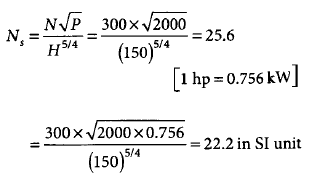Fluid Mechanics, Strength Of Materials - 1 - Question 3

### Match List I with List II and select the correct answer using the codes given below the lists: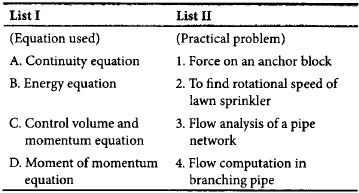Codes: ABCD

Fluid Mechanics, Strength Of Materials - 1 - Question 4

Match List I with List II and select the correct answer using the codes given below the lists: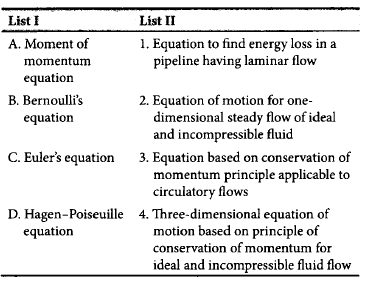Codes:
ABCD

Fluid Mechanics, Strength Of Materials - 1 - Question 5

When two identical centrifugal pumps are operating in series on a common rising main, then

Fluid Mechanics, Strength Of Materials - 1 - Question 6

The stream function of a two dimensional flow is given by ψ = 2xy.  the resultant velocity at the point P(2,3) is

Detailed Solution for Fluid Mechanics, Strength Of Materials - 1 - Question 6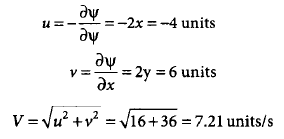Fluid Mechanics, Strength Of Materials - 1 - Question 7

Two long pipes in parallel are used to carry water between two reservoirs. The diameter of one pipe is twice that of the other. Both the pipes have the same value of friction factor. Neglect minor losses. What is the ratio of flow rates through the two pipes?

Fluid Mechanics, Strength Of Materials - 1 - Question 8

The velocity distribution for flow over a plate is given by u = 0.5y - y2, where u is the velocity in m/s at a distance y m above the plate. If the dynamic viscosity of the fluid is 0.9 Ns/m2, then what is the shear stress at 0.20 m from the boundary?

Detailed Solution for Fluid Mechanics, Strength Of Materials - 1 - Question 8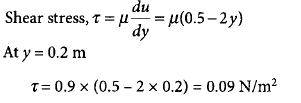Fluid Mechanics, Strength Of Materials - 1 - Question 9

The head loss in a pipe of diameter d carrying oil at a flow rate Q over a distance l is h. The pipe is replaced by another with half the diameter, all other things remaining the same. The head loss in this case will be

Detailed Solution for Fluid Mechanics, Strength Of Materials - 1 - Question 9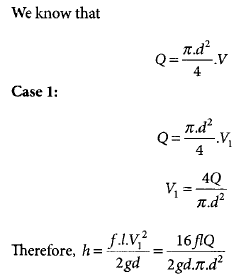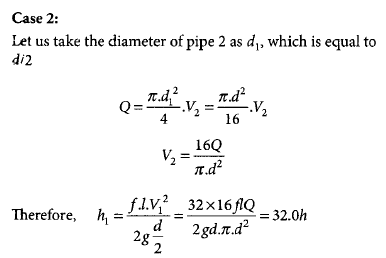*Answer can only contain numeric values
Fluid Mechanics, Strength Of Materials - 1 - Question 10

hydraulic turbine has a discharge of 5 m3/s, when operating under a head of 20 m with a speed of 500 rpm. If it is to operate under a head of 15 m, for the same discharge, the rotational speed in rpm will approximately be

Fluid Mechanics, Strength Of Materials - 1 - Question 11

A cylindrical vessel with a constant plane area of 1 m2 is rotated about its vertical axis such that the liquid inside the vessel is about to spill. If the height of the vessel is 2 m and the height of the paraboloid at 1 m, then the volume (in m3) of the liquid in the vessel will be

Fluid Mechanics, Strength Of Materials - 1 - Question 12

In steady flow of a compressible fluid through a pipe, the density, area, and velocity at a particular section are 1.5 kg/m3, 0.5 m2, and 3 m/s, respectively. At another section, the density and area are 0.75 kg/m3 and 1.0 m2, respectively. What is the velocity at this section?

Detailed Solution for Fluid Mechanics, Strength Of Materials - 1 - Question 12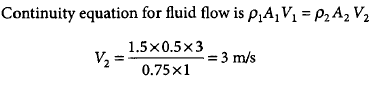Fluid Mechanics, Strength Of Materials - 1 - Question 13

The following statements relate to the pressure exerted by a fluid on a submerged curved surface.
1.The vertical component of hydrostatic force acting on a submerged curved surface acts through the center of volume of the fluid directly above the submerged area.
2.The horizontal component of the force acting on a curved surface is the hydrostatic force acting on the vertical projection of the curved surface.
3.The resultant force on a curved surface acts on the bottom of the curved surface.
Of these statements,

Fluid Mechanics, Strength Of Materials - 1 - Question 14

Match List I with List II and select the correct answer using the codes given below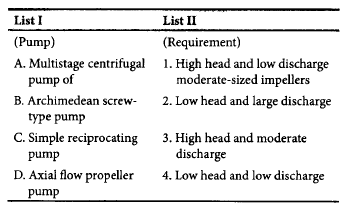Codes  - ABCD

Fluid Mechanics, Strength Of Materials - 1 - Question 15

Consider the following statements relating to hydraulic gradient line and energy gradient line:
1.In the case of a fluid flowing in a pipeline, hydraulic gradient line and energy gradient line may coincide.
2.The line joining the points representing piezometric heads is known as hydraulic gradient.
In the case of ideal fluid, energy gradient line is always horizontal.
3.Hydraulic gradient line has a downward slope in the case of flow through pipes.
Of these statements,

## GATE Mechanical (ME) 2023 Mock Test Series

25 docs|229 tests
 Use Code STAYHOME200 and get INR 200 additional OFF Use Coupon Code
Information about Fluid Mechanics, Strength Of Materials - 1 Page
In this test you can find the Exam questions for Fluid Mechanics, Strength Of Materials - 1 solved & explained in the simplest way possible. Besides giving Questions and answers for Fluid Mechanics, Strength Of Materials - 1, EduRev gives you an ample number of Online tests for practice

## GATE Mechanical (ME) 2023 Mock Test Series

25 docs|229 tests